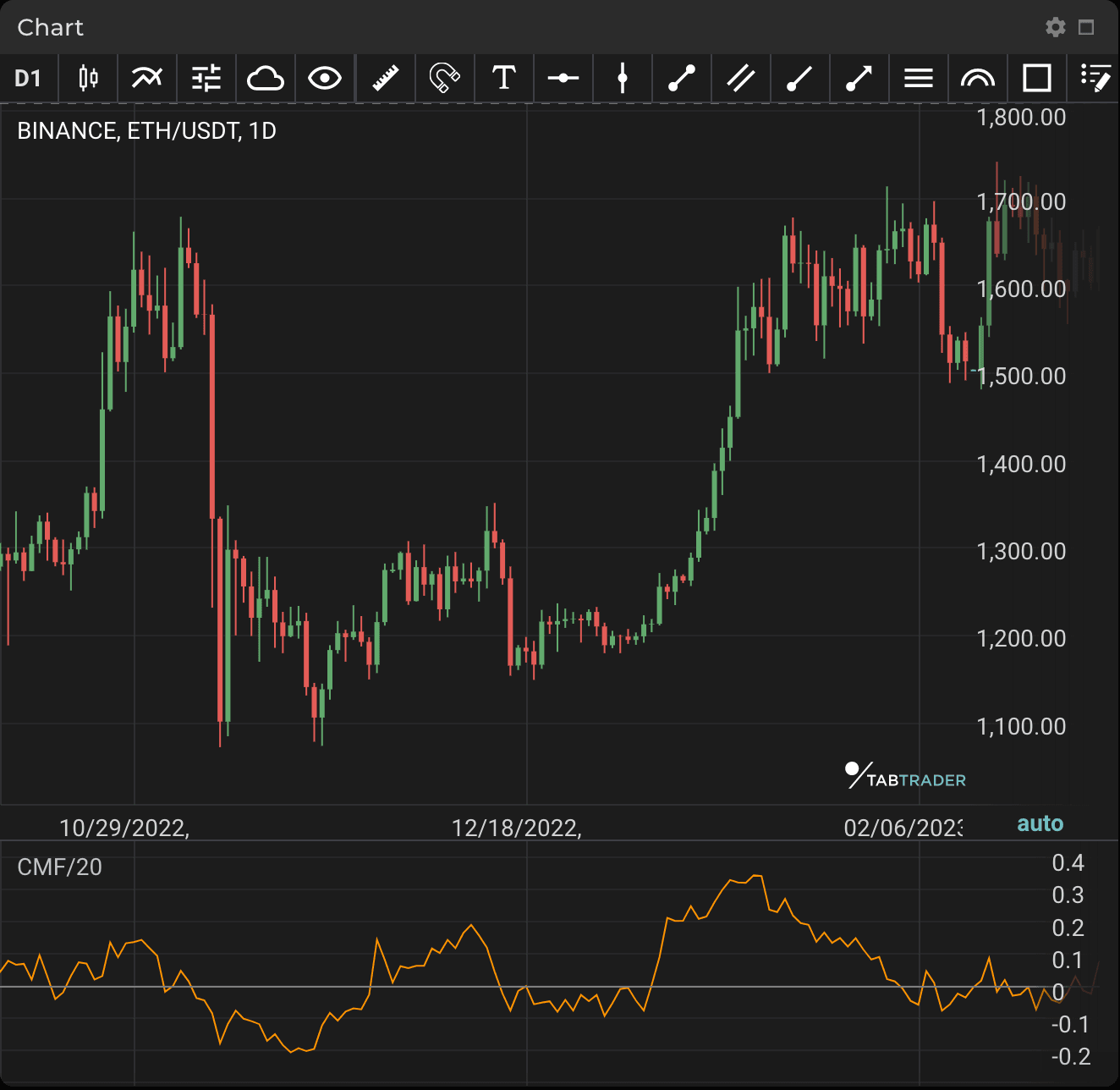# Chaikin Money Flow

Publication date is

Chaikin Money Flow (CMF) is a volume-weighted average of accumulation and distribution over a set period of time. The main principle behind CMF is the nearer the closing price is to the high, the more accumulation has taken place. In contrast, the nearer the closing price is to the low, the more distribution has occurred.

If price action closes above the bar's midpoint on increasing volume, CMF will be positive. Conversely, if price action closes below the bar's midpoint on increasing volume, CMF will be negative.

Essentially, buying pressure is stronger when the indicator is positive and selling pressure is stronger when the indicator is negative. When CMF crosses the zero line (“0” on the scale), this can be an indication of a trend reversal.

Traders usually use CMF in both cases: in a bullish trend, when its value is above 0, continuous buying pressure can indicate that prices will keep rising. In a bearish trend, when CMF values are below 0, continuous selling pressure can indicate that prices will fall further. Note that brief crosses can result in false signals. CMF works well in combination with other indicators, but on its own it can lead to misleading and untimely signals.CMF

### Calculation

CMF is calculated in four steps. The example below takes 20 periods as the default.

1. Money Flow Multiplier = [(Close  -  Low) - (High - Close)] /(High - Low)
2. Money Flow Volume = Money Flow Multiplier x Volume for the Period
3. 20-period CMF = 20-period Sum of Money Flow Volume / 20-period Sum of Volume

Each period's Money Flow Volume depends on the Money Flow Multiplier. This multiplier is positive when the close is in the upper half of the period's high-low range and negative when the close is in the lower half. The multiplier equals 1 when the close equals the high and -1 when the close equals the low. In this way, the multiplier adjusts the amount of volume that ends up in Money Flow Volume. Volume is in effect reduced unless the Money Flow Multiplier is at its extremes (+1 or -1).

Looking to get started in crypto trading?

Try TabTrader on mobile or web!